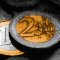Savings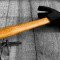DIY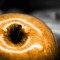Health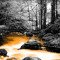EcologyScience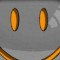Fun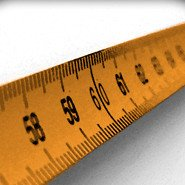# Convert distances

Convert distances with the most commonly used units of measurement. Possible conversions in the metric system, imperial / US system and astronomical system.

km
hm
dam
m
dm
cm
mm
μm
nm

mi
rd
yd
ft
in

pc
ly
au

## Info

In the physical sciences and engineering, when one speaks of units of length, the word length is synonymous with distance. There are several units that are used to measure length. Historically, units of length may have been derived from the lengths of human body parts, the distance traveled in a number of paces, the distance between landmarks or places on the Earth, or arbitrarily on the length of some common object.

In the International System of Units (SI), the basic unit of length is the metre and is now defined in terms of the speed of light. The centimetre and the kilometre, derived from the metre, are also commonly used units. In U.S. customary units, English or Imperial system of units, commonly used units of length are the inch, the foot, the yard, and the mile.

Units used to denote distances in the vastness of space, as in astronomy, are much longer than those typically used on Earth and include the astronomical unit, the light-year, and the parsec. Source : Wikipédia.org